# Quantum mechanics, reductionism, and irreducibility

Take a look at the following two qubit state: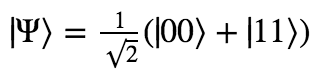Is it possible to describe this two-qubit system in terms of the states of the two individual particles that compose it? The principle of reductionism suggests to us that yes it should be possible; after all, of course we can always take any larger system and describe it perfectly fine in terms of its components.

But this turns out to not be the case! The above state is a perfectly allowable physical configuration of two particles, but there is no accurate description of the state of the individual particles composing the system!

Multi-particle systems cannot in general be reduced to their parts. This is one of the shocking features of quantum mechanics that is extremely easy to prove, but is rarely emphasized in proportion to its importance. We’ll prove it now.

Suppose we have a system composed of two qubits in states |Ψ1⟩ and |Ψ2⟩. In general, we may write:Now, as we’ve seen in previous posts, we can describe the state of the two qubits as a whole by simply smushing them together as follows:So the set of all two-qubit states that can be split in their component parts is the set of all states arising from all possible values of α1, α2, β1, and β2 such that all states are normalized. I.e.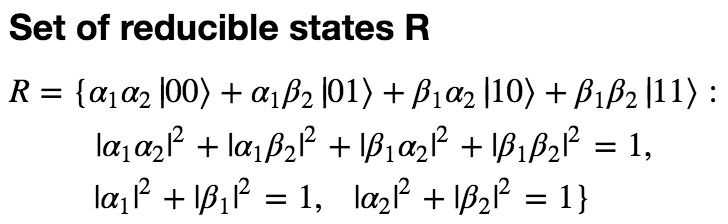However, there’s also a theorem that says that if any two states are physical possible, then all normalized linear combinations are physically possible as well. Because the states |00⟩, |01⟩, |10⟩, and |11⟩ are all physically possible, and because they form a basis for the set of two-qubit states, we can write out the set of all possible states:Now the philosophical question of whether or not there exist states that are irreducible can be formulated as a precise mathematical question: Does A = R?

And the answer is no! It turns out that A is much much larger than R.

The proof of this is very simple. R and A are both sets defined by a set of four complex numbers, and they share a constraint. But R also has two other constraints, independent of the shared constraint. That is, the two additional constraints cannot be derived from the first (try to derive it yourself! Or better, show that it cannot be derived). So the set of states that satisfy the conditions necessary to be in R must be smaller than the set of states that satisfy the conditions necessary to be in A. This is basically just the statement that when you take a set, and then impose a constraint on it, you get a smaller set.

An even simpler proof of the irreducibility of some states is to just give an example. Let’s return to our earlier example of a two-qubit state that cannot be decomposed into its parts:Suppose that ⟩ is reducible. Then for both |00⟩ and |11⟩ to have a nonzero amplitude, there must be a nonzero amplitude for the first qubit to be in the state |0⟩ and for the second to be in the state |1⟩. But then there can’t be zero amplitude for the state |01⟩. Q.E.D.!

More precisely:So here we have a two-qubit state that is fundamentally irreducible. There is literally no possible description of the individual qubits on their own. We can go through all possible states that each qubit might be in, and rule them out one.

Let’s pause for a minute to reflect on how totally insane this is. It is a definitive proof that according to quantum mechanics, reality cannot necessarily be described in terms of its smallest components. This is a serious challenge to the idea of reductionism, and I’m still trying to figure out how to adjust my worldview in response. While the notion of reductionism as “higher-level laws can be derived as approximations of the laws of physics” isn’t challenged by this, the notion that “the whole is always reducible to its parts” has to go.

In fact, I’ll show in the next section that if you try to make predictions about an system but analyze it in terms of its smallest components, you will not in general get the right answer.

# Predictive accuracy requires holism

So suppose that we have two qubits in the state we already introduced:You might think the following: “Look, the two qubits are either both in the state |0, or both in the state |1⟩. There’s a 50% chance of either one happening. Let’s suppose that we are only interested in the first qubit, and don’t care what happens with the second one. Can’t we just say that the first qubit is in a state with amplitudes 1/√2 in both states |0⟩ and |1⟩? After all, this will match the experimental results when we measure the qubit (50% of the time it is |0⟩ and 50% of the time it is |1⟩.”

Okay, but there are two big problems with this. First of all, while it’s true that each particle has a 50% chance of being observed in the state |0⟩, if you model these probabilities as independent of one another, then you will end up concluding that there is a 25% chance of the first particle being in the state |0⟩ and the second being in the state |1⟩. Whereas in fact, this will never happen!

You may reply that this is only a problem if you’re interested in making predictions about the state of the second qubit. If you are solely looking at your single qubit, you can still succeed at predicting what will happen when you measure it.

Well, fine. But the second, more important point is that even if you are able to accurately describe what happens when you measure your single qubit, you can always construct a different experiment you could perform that this same description will give the wrong answer for.

What this comes down to is the observation that quantum gates don’t operate the same way on 1/√2 (|00⟩ + |11⟩) as on 1/√2 (|0⟩ + |1⟩).

Suppose you take your qubit and pretend that the other one doesn’t exist. Then you apply a Hadamard gate to just your qubit and measure it. If you thought that the state was initially 1/√2 (|0⟩ + |1⟩), you will now think that your qubit is in the state |0⟩. You will predict with 100% confidence that if you measure it now, you will observe |0⟩.

But in fact when you measure it, you will find that 50% of the time it is |0⟩ and 50% of the time it is |1⟩! Where did you go wrong? You went wrong by trying to describe the particle as an individual entity.

Let’s prove this. First we’ll figure out what it looks like when we apply a Hadamard gate to only the first qubit, in the two-qubit representation: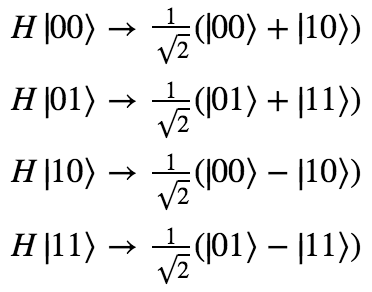So we have a ​25% chance of observing each of |00⟩|10⟩|01⟩, and|11⟩. Looking at just your own qubit, then, you have a 50% chance of observing |0⟩ and a 50% chance of observing |1⟩.

While your single-qubit description told you to predict a 100% chance of observing |0⟩, you actually would get a 50% chance of |0⟩ and a 50% chance of |1⟩.

Okay, but maybe the problem was that we were just using the wrong amplitude distribution for our single qubit. There are many choices we could have made for the amplitudes besides 1/√2 that would have kept the probabilities 50/50. Maybe one of these correctly simulates the behavior of the qubit in response to a quantum gate?

But no. It turns out that even though it is correct that there is a 50/50 chance of observing the qubit to be |0⟩ or |1⟩, there is no amplitude distribution matching this probability distribution that will correctly predict the results of all possible experiments.

Quick proof: We can describe a general two-qubit state with a 50/50 probability of being observed in |0⟩ and |1⟩ as follows: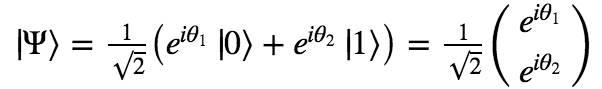For any ⟩, we can construct a specially designed quantum gate U that transforms ⟩ into |0⟩:Applying U to our single qubit, we now expect to observe |0⟩ with 100% probability. But now let’s look at what happens if we consider the state of the combined system. The operation of applying U to only the first qubit is represented by taking the tensor product of U with the identity matrix I: U ⊗ I.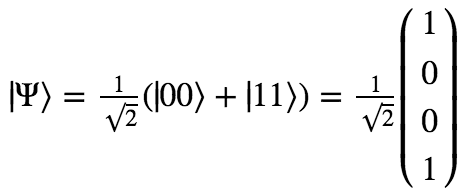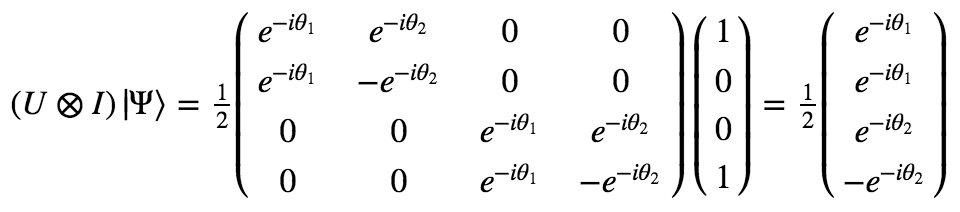What we see is that the two-qubit state ends up with a 25% chance of being observed as each of |00⟩, |01⟩, |10⟩, and |11⟩. This means that there is still a 50% chance of the first qubit being observed as |0⟩ and |1⟩.

This means that for every possible single qubit description of the first qubit, we can construct an experiment that will give different results than the model predicts. And the only model that always gives the right experimental predictions is a model that considers the two qubits as a single unit, irreducible and impossible to describe independently.

To recap: The lesson here is that for some quantum systems, if you describe them in terms of their parts instead of as a whole, you will necessarily make the wrong predictions about experimental results. And if you describe them as a whole, you will get the predictions spot on.

# So how many states are irreducible?

Said another way, how much larger is A (the set of all states) than R (the set of reducible states)? Well, they’re both infinite sets with the same cardinality (they each have the cardinality of the continuum, |ℝ|). So in this sense, they’re the same size of infinity. But we can think about this by considering the dimensionality of these various spaces.

Let’s take another look at the definitions of A and R:Each set is defined by four complex numbers, or 8 real numbers. If we ignored all constraints, then, our sets would be isomorphic to ℝ8.

Now, each share the same first constraint, which says that the overall state must be normalized. This constraint cuts one dimension off of the space of solutions, making it isomorphic to ℝ7.

That’s the only constraint for A, so we can say that A ~ ℝ7. But R involves two further constraints (the normalization conditions for each individual qubit). So we have three total constraints. However, it turns out that one of them can be derived from the others – two normalized qubits, when smushed together, always produce a normalized state. This gives us a net two constraints, meaning that the space of reducible states is isomorphic to ℝ6.

The space of irreducible states is what’s left when we subtract all elements of R from A. The dimensionality of this is just the same as the dimensionality of A. (A 3D volume minus a plane is still a 3D volume, a plane minus a curve is still two dimensional, a curve minus a point is still one dimensional, and so on.)

So both the space of total states and the space of irreducible states are 7-real-dimensional, while the space of reducible states is 6-real dimensional.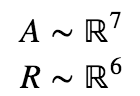You can visualize this as the space of all states being a volume, through which cuts a plane that composes all reducible states. The entire rest of the volume is the set of irreducible states. Clearly there are a lot more irreducible states than reducible states.

What about if we consider totally reducible three-qubit states? Now things are slightly different.

The set of all possible three qubit states (which we’ll denote A3) is a set of 8 complex numbers (16 real numbers) with one normalization constraint. So A3 ~ ℝ15.

The set of all totally reducible three qubit states (which we’ll denote R3) is a set of only six complex numbers. Why? Because we only need to specify two complex numbers for each of the three individual qubits that will be smushed together. So we start off with only 12 real numbers. Then we have three constraints, one for the normalization of each individual qubit. And the final normalization constraint (of the entire system) follows from the previous three constraints. In the end, we see that R3 ~ ℝ9.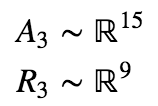Now the space of reducible states is six-dimensions less than the space of all states.

How does this scale for larger quantum systems? Let’s look in general at a system of N qubits.

AN is a set of 2N complex amplitudes (2N+1 real numbers), one for each N qubit state. There is just one normalization constraint. Thus we have a space with 2N+1 – 1 real dimensions.

On the other hand, RN is a set of only 2N complex amplitudes (4N real numbers), two for each of the N individual qubits. And there are N independent constraints ensuring that all states are normalized. So we have: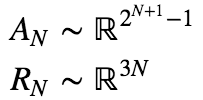The point of all of this is that as you consider larger and larger quantum systems, the dimensionality of the space of irreducible states grows exponentially, while the dimensionality of the space of reducible states only grows linearly. If we were to imagine randomly selecting a 20-qubit state from the space of all possibilities, we would be exponentially more likely to ending up with a space that cannot be described as a product of each of its parts.

What this means is that irreducibility is not a strange exotic phenomenon that we shouldn’t expect to see in the real world. Instead, we should expect that basically all systems we’re surrounded by are irreducible. And therefore, we should expect that the world as a whole is almost certainly not describable as the sum of individual parts.

## 2 thoughts on “Quantum mechanics, reductionism, and irreducibility”

1. Pingback: Rising Entropy